# Test: Class 12 Chemistry: CBSE Sample Question Paper- Term I (2021-22)

## 55 Questions MCQ Test Sample Papers for Class 12 Medical and Non-Medical | Test: Class 12 Chemistry: CBSE Sample Question Paper- Term I (2021-22)

Description
Attempt Test: Class 12 Chemistry: CBSE Sample Question Paper- Term I (2021-22) | 55 questions in 90 minutes | Mock test for Class 12 preparation | Free important questions MCQ to study Sample Papers for Class 12 Medical and Non-Medical for Class 12 Exam | Download free PDF with solutions
QUESTION: 1

### The IUPAC name of isobutyl alcohol is :

Solution:

The structural formula of isobutyl alcohol is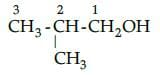IUPAC name : 2 methyl propan-1-ol

QUESTION: 2

### Formation of ortho hydroxy benzoic acid from phenol using sodium hydroxide is:

Solution:

Phenoxide ion generated by treating phenol with sodium hydroxide. Further it undergoes electrophilic substitution with carbon dioxide to form ortho hydroxybenzoic acid as the main reaction product.

QUESTION: 3

### Glucose and fructose are prepared in equal amount when:

Solution:

It is a method of preparation of glucose form sucrose i.e. cane sugar.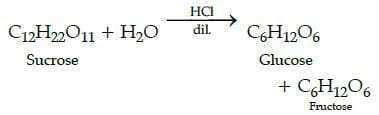QUESTION: 4

Which is true for crystalline solids:

Solution:

The other three are characteristics of isotropic solids.

QUESTION: 5

All the elements of group 15 form EX3 and EX5 except:

Solution:

Nitrogen does not form pentahalide due to non-availability of the d orbitals in its valence shell.

QUESTION: 6

When constituent particles are present only on the corner positions of a unit cell:

Solution:

The others are the type of centred unit cell which have one or more constituent particles present at positions other than corners in addition to those at corners.

QUESTION: 7

Chloroform mixed with nitrogen gas is a type of which solution:

Solution:

Chloroform mixed with nitrogen gas is an example of Liquid + gas solution.

QUESTION: 8

What is incorrect about fibrous proteins:

Solution:

It is a characteristic of globular protein.

QUESTION: 9

Name the following structures of proteins: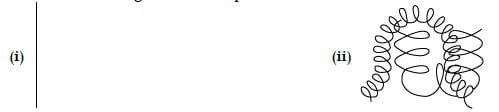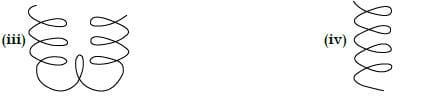Solution:

The structure is -primary, quaternary, tertiary and secondary.

QUESTION: 10

Which of the following is not a haloalkane ?

Solution:

2-Chloro acetophenone is a substitute of acetophenone. It is phenacyl Chloride and use as a tear gas.

QUESTION: 11

In solid alkali halides, the appearance of colour is due to:

Solution:

The colour is due to excitation of electrons (at anionic site) when they absorb energy from the visible light falling on the crystals.

QUESTION: 12

Supersonic jet aeroplanes are responsible for depleting the conc. of ozone layer because:

Solution:

Nitrogen oxides emitted from the exhaust systems of supersonic jet aeroplanes is slowly depleting the concentration of the ozone layer in the upper atmosphere as nitrogen oxides (particularly nitrogen monoxide) combine very rapidly with ozone.

QUESTION: 13

Which of the following does not react with oxygen directly ?

Solution:

Platinum (Pt) is an inert metal and does not react very easily. All other elements, Zn, Ti and Fe, are quite reactive.
Hence, Pt does not react with oxygen directly.

QUESTION: 14

In ______the domains are oppositely oriented and cancel out each other’s magnetic moment :

Solution:

MnO is diamagnetic in nature.

QUESTION: 15

Which of the following is not a chiral compound :

Solution:

It does not contain asymmetric carbon atom.

QUESTION: 16

For any solution the partial vapour pressure of each volatile component in the solution is directlyproportional to its_______________.

Solution:

According to Raoult’s law, for any solution the partial vapour pressure of each volatile component in the solution is directly proportional to its mole fraction.

QUESTION: 17

The value of van’t Hoff factors for K2SO4, NaCl and KCl is :

Solution:

K2SO4 ionizes to give 2K+ and
SO4 - ions (total 3 ions). NaCl gives Na+ and
Cl- ions(total 2 ions) KCl gives K+ and Clions
(total 2 ions).

QUESTION: 18

Many wet gases are dried by passing through sulphuric acid, this shows sulphuric acid is:

Solution:

Sulphuric acid has strong affinity for water as moisture in gases that act as a strong dehydrating agent.

QUESTION: 19

Choose the molecules in which carbon atom marked with asterisk (*) is asymmetric ?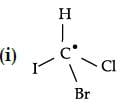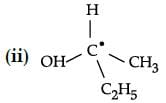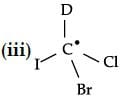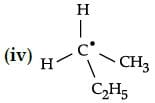Solution:

Asymmetric carbon atoms are those which have four different groups attached to carbon atom.

QUESTION: 20

Calculate the number of atoms per unit cell of iron crystal lattice if the edge length is 268 pm anddensity is 7. 86 g / cm3: [Molar mass of Fe = 56 g / mol].

Solution:

a = 268 pm = 268x10-10 cm
d = 7.86 g cm-3
M =56g m of-1
NA = 6.022×1023 mol-1
n = ?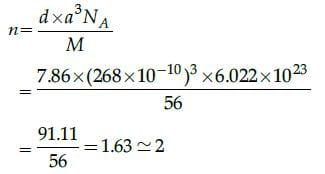QUESTION: 21

How much amount of NaCl in gram will be required for the preparation of its 5,6 w/v 1000 mlaqueous solution ?

Solution: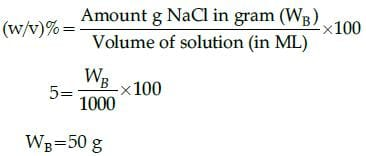QUESTION: 22

Fuming nitric acid is a mixture of:

Solution:

Fuming acid =HNO3 + NO2

QUESTION: 23

The presence of halogen atom in the haloarenes directs the incoming substituent in electrophilessubstitution reactions to:

Solution: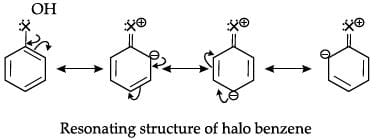∴ Electron density is highest at ortho and para positions, so, halogen atom (x) directs the incoming substituent in electrophilic substitution reaction to ortho and para positions.

QUESTION: 24

Give the major product in the following reaction:

C2H5Br + alc. AgCN → Product

Solution: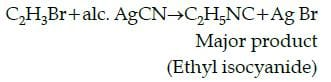QUESTION: 25

Glucose is converted into ethanol in the persence of:

Solution: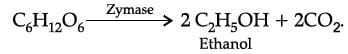QUESTION: 26

Which allotropic form of sulphur is most stable at room temperature:

Solution:

Rhombic sulphur consists of 8 sulphur atoms arranged in an octahedral shape which form the most stable structure that doesn’t change gradually.

QUESTION: 27

The number of sulphur molecules in monoclinic and rhombic sulphur:

Solution:

Both rhombic and mono clinic sulphur have S8 molecules which is the most allotropic form.

QUESTION: 28

Sulphur dioxide when passed through water forms:

Solution:

SO2(g) + H2O(l) ⇋ H2SO3(l)

QUESTION: 29

Choose the correct answer from the options given: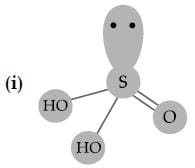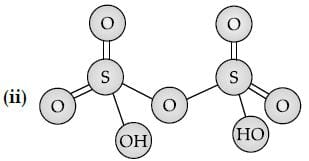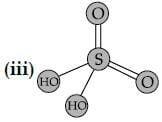Solution:

The given structure (i), (ii) and (iii) is showing Sulphurous acid, pyro sulphuric acid, sulphuric acid respectively.

QUESTION: 30

Sulphuric acid is used to manufacture more volatile acids from their corresponding salts due to its:

Solution:

Sulphuric acid have high boiling point and low volatility that combines with salt like NaCl to form volatile acids like HCl and HNO3.

QUESTION: 31

In the complex ion [AuXe4]2+, Xe acts as:

Solution:

Xe acts as a central atom in the complex [AuXe4]2+

QUESTION: 32

Hybridisation shown by Au in [AuXe4]2+ is:

Solution:

sp3d hybridization produces liner geometry
Hybridisation = 1/2 [No. of electrons in the value shell of atom + No. of monovalant atoms surrounding the atom - charge on cation + change on anion]

QUESTION: 33

Compounds of noble gases except _______ are known.

Solution:

Helium has complete last shell. It does not react with any other element to form compound.

QUESTION: 34

Xe is a ___________ ligand.

Solution:

As it can donate single pair of electrons from one atom.

QUESTION: 35

In the formation of complex entity, the central atom acts as:

Solution:

As it accepts pair of electrons from the ligand, thus forming coordinate bond.

QUESTION: 36

SN2 mechanism is not favoured when:

Solution:

When an electrophile is attached to allylic, tertiary or benzylic carbon SN1  reaction is favoured.

QUESTION: 37

Which of the following is likely to proceed with SN1 reaction:

Solution:

Electrophile at tertiary carbon, weak nucleophile and protic solvent.

QUESTION: 38

Which of the following factors does not have any significant effect on solubility of solids in liquids ?

Solution:

Pressure does not have any significant effect on solubility of solids in liquids. As solids and liquids are highly incompressible and thus they remain unaffected by changes in pressure.

QUESTION: 39

Which of the following methods is must suitable for determination of molecular mass of polymers ?

Solution:

Molecular mass of polymers can be calculated using osmotic pressure method. This is because the values given by other colligative properties are so low that they cannot be measured.

QUESTION: 40

Mark the correct statement.

Solution:

Fluorine is a stronger oxidising agent than chlorine due to low enthalpy of dissociation of F-F bond.

QUESTION: 41

The acid strength of tertiary alcohol is much lesser than that of primary alcohol. It is due to presence of:

Solution:

An electron-releasing group (–CH3, –C2H5) increases electron density on oxygen tending to decrease the polarity of O-H bond which leads to decrease in acid strength.

QUESTION: 42

The increasing order of boiling point of haloalkenes is:

Solution:

The boiling point of haloalkanes increases in following order:

RCl < RBr < RI

It is due to increases in size and mass of the halogen atom by which the magnitude of van der waal forces of attraction increases and boiling point of haloalkane increases.

QUESTION: 43

Ethanal undergoes reduction with LiAlH4 to produce:

Solution: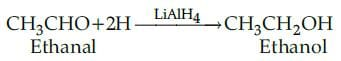QUESTION: 44

The number of hydrogen bonds between cytosine and guanine is:

Solution:

Cytosine(C) pairs with guanine (G) through three hydrogen bonds.

C ≡ G

QUESTION: 45

Given below are two statements labelled as Assertion (A) and Reason (R) and the question give the following line select the must appropriate answers from the options given below:

Assertion (A): A body-centred cubic (bcc) unit cell has an atom at each of its corners and also one atom at its body centre.
Reason (R): There are two atoms per unit cell.

Solution:

No. of atoms = 8 corners x 1/8 per corner atom = 8 x 1/8 = 1 atom

QUESTION: 46

Direction: Given below are two statements labelled as Assertion (A) and Reason (R) and the question give the following line select the must appropriate answers from the options given below:

Assertion (A): Salmon should be included in diet.
Reason (R): Tryptophan is an essential amino acid.

Solution:

Salmon help body to produce serotonin. Salmon contains amino acid tryptophan, which helps to produce more serotonin. Essential amino acids need to be taken by diet.

QUESTION: 47

Direction: Given below are two statements labelled as Assertion (A) and Reason (R) and the question give the following line select the must appropriate answers from the options given below:

Assertion (A): Nitrogen exists as diatomic molecule with a triple bond.
Reason (R): Phosphorus and arsenic form single bonds.

Solution:

Nitrogen has the unique ability to form pπ-pπ with itself. Thus, it forms triple bond (one s and two p orbitals).

QUESTION: 48

Direction: Given below are two statements labelled as Assertion (A) and Reason (R) and the question give the following line select the must appropriate answers from the options given below:

Assertion (A): Ethanol mixed with methanol is called as denatured alcohol.
Reason (R): Methanol is oxidised to methanal and then to methanoic acid.

Solution:

Ethanol mixed with methanol is called as denatured alcohol.
Denatured alcohol is harmful to body. It may cause blindness or death in some cases.

QUESTION: 49

Direction: Given below are two statements labelled as Assertion (A) and Reason (R) and the question give the following line select the must appropriate answers from the options given below:

Assertion (A): Wherever a sphere of the second layer is above the void of the first layer (or vice versa)
a tetrahedral void is formed.
Reason (R): The spheres of the third layer are exactly aligned with those of the first layer.

Solution:

Tetrahedral voids are formed because a tetrahedron is formed when the centres of these four spheres are joined.

QUESTION: 50

Match the following: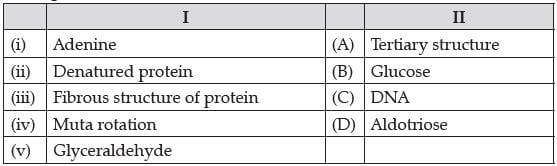Which of the following is the best matched options ?

Solution:

Adenine is a purine base found in DNA. Fibrous structure of protein-Tertiary structure. Tertiary structure of proteins are fibrous structure and globular structure-Mutarotation-Glucose

The process which involes the change in the optical rotation of either form of glucose in aqueous solutions to that of equilibrium mixture is called mutarotation.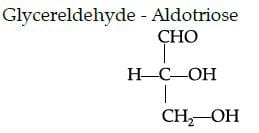It is a monosaccharide having three carbon atoms and aldehyde group, so it is an example of aldotriose.

QUESTION: 51

Which of the following analogies is correct:

Solution:

For BCC (Body centred Cubic
unit call)
Number of atoms per unit cell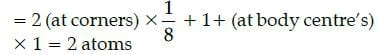For FCC (Face centred cubic unit cell) Number of atoms per unit cell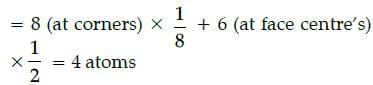QUESTION: 52

Complete the following analogy:Number of moles of solute per litre of solution: A:: Number of moles of solute per kilogram of solvent: B

Solution:

The number of moles of solute per litre of solution is called molarity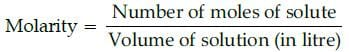The number of moles of solute per kilogram of solvent is called molality.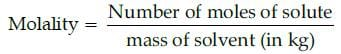QUESTION: 53

The chief sources of proteins are milk, cheese, pulses, peanuts, fish, meat, etc. They occur in every part of the body and form the fundamental basis of structure and functions of life. They are also required for growth and maintenance of body. The word protein is derived from Greek word, “proteios” which means primary or of prime importance.

Amino acids contain amino (–NH2) and carboxyl (–COOH) functional groups. Depending upon the relative position of amino group with respect to carboxyl group, the amino acids can be classified as α, β, γ, δ and so on.

Name the most abundant bio molecule of the living system:

Solution:

Proteins occur in every part of the body and from the fundamental basis of structure and functions of life. So, it is most abundant biomolecule of the living system .

QUESTION: 54

The chief sources of proteins are milk, cheese, pulses, peanuts, fish, meat, etc. They occur in every part of the body and form the fundamental basis of structure and functions of life. They are also required for growth and maintenance of body. The word protein is derived from Greek word, “proteios” which means primary or of prime importance.

Amino acids contain amino (–NH2) and carboxyl (–COOH) functional groups. Depending upon the relative position of amino group with respect to carboxyl group, the amino acids can be classified as α, β, γ, δ and so on.

Choose the correct function of proteins:

Solution:

Proteins are required for regulation of body tissues and organs.

QUESTION: 55Use Code STAYHOME200 and get INR 200 additional OFF Use Coupon Code